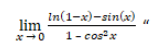“On October 3rd he asked me what day it was… it’s October 3rd.” And just like that, Mean Girls Day was born. Whilst not an official national holiday (yet...) the celebrations that take place to mark the occasion over the years have become increasingly extravagant; themed club nights, outdoor cinemas and transforming a leisure centre in London into a full-scale copy of North Shore High School.

Don’t be put off by mainstream early 00s’ popular culture – maths is key feature of the film too! The protagonist Cady is a keen mathematician and even competes on the school’s quiz team – the Mathletes (albeit after first rejecting them due to social pressure). To celebrate Mean Girls Day, as well as rewatching the cult classic and wearing some pink on Wednesday, we’ve found those tricky questions from the ‘Illinois High School Mathletes State Championship’ to check the maths and see if we could match up to the hero of the story’s abilities!

Question 1

“Twice the larger of two numbers is three more than five times the smaller and the sum of four times the larger and three times the smaller is 71. What are the numbers?”

Overwhelming to process in the film but translating into simultaneous equations suddenly becomes easily solvable! Let 𝑥<𝑦 be the two numbers in question. Then

2𝑦=3+5𝑥
4𝑦+3𝑥=71

From here it is simple to rearrange and solve, so as Kevin says 𝑥=5 and 𝑦=14.

Question 2

“Find an odd three digit number whose digits add up to 12. The digits are all different and the difference between the first two digits equals the difference between [the last two digits].”

The answer given by ‘Kratzer from Marymount Prep’ is 741, a correct answer; but are there others? We are searching for three integers say 𝑎,𝑏,𝑐 such that

1. 𝑐 is odd – (𝑎𝑏𝑐 is a three digit odd number)

2. 𝑎+𝑏+𝑐=12

3. |𝑏–𝑎|=|𝑐–𝑏|

4. 𝑎≠𝑏≠𝑐

If 𝑐=9 then 𝑎+𝑏=3, and so it would be impossible for condition 3 to hold.

Taking 𝑐=7 then 𝑎+𝑏=5 for condition 2 to hold. For 3 to also be respected then 𝑎=1 and 𝑏=4, flipping the answer given in the film.

Now considering 𝑐=5, then it is quick to see the only solution is for 𝑎=3 and 𝑏=4. And for 𝑐 = 3, it must be that 𝑎=5 and 𝑏=4. Finally if 𝑐 = 1 then the options for a and b are 7 and 4, the answer given in the film.

Therefore the possible answers are 147, 345, 543 and 741, easy when you have the time, so don’t be too hard on yourself Cady (“Shoot I was really rusty”).

Question 3

And finally the competition winning question!

“Find the limit of this equation,One way to solve is to consider the power series approximations for close to zero for each term. The limit becomes approximated toApproaching from above zero, the limit will tend to negative infinity. However, from below it will tend to positive infinity and so as Cady says “the limit does not exist”!

Maths in the media..

Understanding some beautiful geometry…